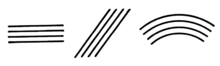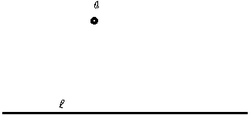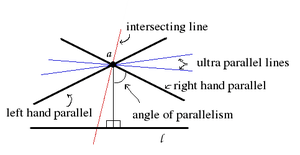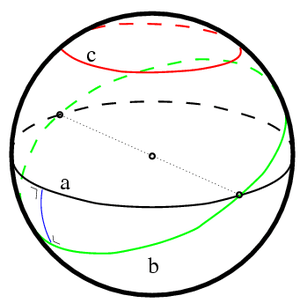# Parallel (geometry)

"Parallel lines" redirects here. For other uses, see Parallel lines (disambiguation).Line art drawing of parallel lines and curves.

In geometry, parallel lines are lines in a plane which do not meet; that is, two lines in a plane that do not intersect or touch each other at any point are said to be parallel. By extension, a line and a plane, or two planes, in three-dimensional Euclidean space that do not share a point are said to be parallel. However, two lines in three-dimensional space which do not meet must be in a common plane to be considered parallel; otherwise they are called skew lines. Parallel planes are planes in the same three-dimensional space that never meet.

Parallel lines are the subject of Euclid's parallel postulate. Parallelism is primarily a property of affine geometries and Euclidean space is a special instance of this type of geometry. Some other spaces, such as hyperbolic space, have analogous properties that are sometimes referred to as parallelism.

## Symbol

The parallel symbol is. For example,indicates that line AB is parallel to line CD.

In the Unicode character set, the "parallel" and "not parallel" signs have codepoints U+2225 (∥) and U+2226 (∦), respectively. In addition, U+22D5 (⋕) represents the relation "equal and parallel to".

## Euclidean parallelism

### Two lines in a plane

#### Conditions for parallelismAs shown by the tick marks, lines a and b are parallel. This can be proved because the transversal t produces congruent corresponding angles, shown here both to the right of the transversal, one above and adjacent to line a and the other above and adjacent to line b.

Given parallel straight lines l and m in Euclidean space, the following properties are equivalent:

1. Every point on line m is located at exactly the same (minimum) distance from line l (equidistant lines).
2. Line m is in the same plane as line l but does not intersect l (recall that lines extend to infinity in either direction).
3. When lines m and l are both intersected by a third straight line (a transversal) in the same plane, the corresponding angles of intersection with the transversal are congruent.

Since these are equivalent properties, any one of them could be taken as the definition of parallel lines in Euclidean space, but the first and third properties involve measurement, and so, are "more complicated" than the second. Thus, the second property is the one usually chosen as the defining property of parallel lines in Euclidean geometry. The other properties are then consequences of Euclid's Parallel Postulate. Another property that also involves measurement is that lines parallel to each other have the same gradient (slope).

#### History

The definition of parallel lines as a pair of straight lines in a plane which do not meet appears as Definition 23 in Book I of Euclid's Elements. Alternative definitions were discussed by other Greeks, often as part of an attempt to prove the parallel postulate. Proclus attributes a definition of parallel lines as equidistant lines to Posidonius and quotes Geminus in a similar vein. Simplicius also mentions Posidonius' definition as well as its modification by the philosopher Aganis.

At the end of the nineteenth century, in England, Euclid's Elements was still the standard textbook in secondary schools. The traditional treatment of geometry was being pressured to change by the new developments in projective geometry and non-Euclidean geometry, so several new textbooks for the teaching of geometry were written at this time. A major difference between these reform texts, both between themselves and between them and Euclid, is the treatment of parallel lines. These reform texts were not without their critics and one of them, Charles Dodgson (a.k.a. Lewis Carroll), wrote a play, Euclid and His Modern Rivals, in which these texts are lambasted.

One of the early reform textbooks was James Maurice Wilson's Elementary Geometry of 1868. Wilson based his definition of parallel lines on the primitive notion of direction. According to Wilhelm Killing the idea may be traced back to Leibniz. Wilson, without defining direction since it is a primitive, uses the term in other definitions such as his sixth definition, "Two straight lines that meet one another have different directions, and the difference of their directions is the angle between them." Wilson (1868, p. 2) In definition 15 he introduces parallel lines in this way; "Straight lines which have the same direction, but are not parts of the same straight line, are called parallel lines." Wilson (1868, p. 12) Augustus De Morgan reviewed this text and declared it a failure, primarily on the basis of this definition and the way Wilson used it to prove things about parallel lines. Dodgson also devotes a large section of his play (Act II, Scene VI § 1) to denouncing Wilson's treatment of parallels. Wilson edited this concept out of the third and higher editions of his text.

Other properties, proposed by other reformers, used as replacements for the definition of parallel lines, did not fare much better. The main difficulty, as pointed out by Dodgson, was that to use them in this way required additional axioms to be added to the system. The equidistant line definition of Posidonius, expounded by Francis Cuthbertson in his 1874 text Euclidean Geometry suffers from the problem that the points that are found at a fixed given distance on one side of a straight line must be shown to form a straight line. This can not be proved and must be assumed to be true. The corresponding angles formed by a transversal property, used by W. D. Cooley in his 1860 text, The Elements of Geometry, simplified and explained requires a proof of the fact that if one transversal meets a pair of lines in congruent corresponding angles then all transversals must do so. Again, a new axiom is needed to justify this statement.

#### Construction

The three properties above lead to three different methods of construction of parallel lines.The problem: Draw a line through a parallel to l.

#### Distance between two parallel lines

Because parallel lines in a Euclidean plane are equidistant there is a unique distance between the two parallel lines. Given the equations of two non-vertical, non-horizontal parallel lines,the distance between the two lines can be found by locating two points (one on each line) that lie on a common perpendicular to the parallel lines and calculating the distance between them. Since the lines have slope m, a common perpendicular would have slope −1/m and we can take the line with equation y = −x/m as a common perpendicular. Solve the linear systemsandto get the coordinates of the points. The solutions to the linear systems are the pointsandThese formulas still give the correct point coordinates even if the parallel lines are horizontal (i.e., m = 0). The distance between the points iswhich reduces toWhen the lines are given by the general form of the equation of a line (horizontal and vertical lines are included):their distance can be expressed as### Two lines in three-dimensional space

Two lines in the same three-dimensional space that do not intersect need not be parallel. Only if they are in a common plane are they called parallel; otherwise they are called skew lines.

Two distinct lines l and m in three-dimensional space are parallel if and only if the distance from a point P on line m to the nearest point on line l is independent of the location of P on line m. This never holds for skew lines.

### A line and a plane

A line m and a plane q in three-dimensional space, the line not lying in that plane, are parallel if and only if they do not intersect.

Equivalently, they are parallel if and only if the distance from a point P on line m to the nearest point in plane q is independent of the location of P on line m.

### Two planes

Similar to the fact that parallel lines must be located in the same plane, parallel planes must be situated in the same three-dimensional space and contain no point in common.

Two distinct planes q and r are parallel if and only if the distance from a point P in plane q to the nearest point in plane r is independent of the location of P in plane q. This will never hold if the two planes are not in the same three-dimensional space.

## Extension to non-Euclidean geometry

In non-Euclidean geometry, it is more common to talk about geodesics than (straight) lines. A geodesic is the shortest path between two points in a given geometry. In physics this may be interpreted as the path that a particle follows if no force is applied to it. In non-Euclidean geometry (elliptic or hyperbolic geometry) the three Euclidean properties mentioned above are not equivalent and only the second one,(Line m is in the same plane as line l but does not intersect l ) since it involves no measurements is useful in non-Euclidean geometries. In general geometry the three properties above give three different types of curves, equidistant curves, parallel geodesics and geodesics sharing a common perpendicular, respectively.

### Hyperbolic geometryIntersecting, parallel and ultra parallel lines through a with respect to l in the hyperbolic plane. The parallel lines appear to intersect l just off the image. This is just an artifact of the visualisation. On a real hyperbolic plane the lines will get closer to each other and 'meet' in infinity.

While in Euclidean geometry two geodesics can either intersect or be parallel, in hyperbolic geometry, there are three possibilities. Two geodesics belonging to the same plane can either be:

1. intersecting, if they intersect in a common point in the plane,
2. parallel, if they do not intersect in the plane, but converge to a common limit point at infinity (ideal point), or
3. ultra parallel, if they do not have a common limit point at infinity.

In the literature ultra parallel geodesics are often called non-intersecting. Geodesics intersecting at infinity are called limiting parallel.

As in the illustration through a point a not on line l there are two limiting parallel lines, one for each direction ideal point of line l. They separate the lines intersecting line l and those that are ultra parallel to line l.

Ultra parallel lines have single common perpendicular (ultraparallel theorem), and diverge on both sides of this common perpendicular.

### Spherical or elliptic geometryOn the sphere there is no such thing as a parallel line. Line a is a great circle, the equivalent of a straight line in spherical geometry. Line c is equidistant to line a but is not a great circle. It is a parallel of latitude. Line b is another geodesic which intersects a in two antipodal points. They share two common perpendiculars (one shown in blue).

In spherical geometry, all geodesics are great circles. Great circles divide the sphere in two equal hemispheres and all great circles intersect each other. Thus, there are no parallel geodesics to a given geodesic, as all geodesics intersect. Equidistant curves on the sphere are called parallels of latitude analogous to the latitude lines on a globe. Parallels of latitude can be generated by the intersection of the sphere with a plane parallel to a plane through the center of the sphere.

## Reflexive variant

In synthetic, affine geometry the relation of two parallel lines is a fundamental concept that is modified from the usage in Euclidean geometry. It is clear that the relation of parallelism is a symmetric relation and a transitive relation. These are two properties of an equivalence relation. In Euclidean geometry a line is not considered to be parallel to itself, but in affine geometry it is convenient to hold a line as parallel to itself, thus yielding parallelism as an equivalence relation.

Another way of describing this type of parallelism is the requirement that their intersection is not a singleton. Two lines are then parallel when they have all or none of their points in common. It has been noted that Playfair's axiom used in affine and Euclidean geometry is then equivalent to the statement that parallelism forms a transitive relation on the set of lines in the plane.

## Notes

1. Although this postulate only refers to when lines meet, it is needed to prove the uniqueness of parallel lines in the sense of Playfair's axiom.
2. "Mathematical Operators – Unicode Consortium" (PDF). Retrieved 2013-04-21.
3. Wylie, Jr. 1964, pp. 92—94
4. Heath 1956, pp. 190–194
5. Richards 1988, Chap. 4: Euclid and the English Schoolchild. pp. 161–200
6. Carroll, Lewis (2009) , Euclid and His Modern Rivals, Barnes & Noble, ISBN 978-1-4351-2348-9
7. Wilson 1868
8. Einführung in die Grundlagen der Geometrie, I, p. 5
9. Heath 1956, p. 194
10. Richards 1988, pp. 180–184
11. Heath 1956, p. 194
12. Only the third is a straightedge and compass construction, the first two are infinitary processes (they require an "infinite number of steps".)
13. H. S. M. Coxeter (1961) Introduction to Geometry, p 192, John Wiley & Sons
14. Wanda Szmielew (1983) From Affine to Euclidean Geometry, p 17, D. Reidel ISBN 90-277-1243-3
15. Andy Liu (2011) "Is parallelism an equivalence relation?", The College Mathematics Journal 42(5):372

## References

• Heath, Thomas L. (1956), The Thirteen Books of Euclid's Elements (2nd ed. [Facsimile. Original publication: Cambridge University Press, 1925] ed.), New York: Dover Publications
(3 vols.): ISBN 0-486-60088-2 (vol. 1), ISBN 0-486-60089-0 (vol. 2), ISBN 0-486-60090-4 (vol. 3). Heath's authoritative translation plus extensive historical research and detailed commentary throughout the text.
• Richards, Joan L. (1988), Mathematical Visions: The Pursuit of Geometry in Victorian England, Boston: Academic Press, ISBN 0-12-587445-6
• Wilson, James Maurice (1868), Elementary Geometry (1st ed.), London: Macmillan and Co.
• Wylie, Jr., C.R. (1964), Foundations of Geometry, McGraw–Hill